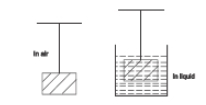A tube of length L is filled completely with an incompressible liquid of mass M and closed at both ends. The tube is then rotated in a horizontal plane about one of its ends with a uniform angular velocity $\omega$. The force exerted by liquid at the other end is

1.  $\frac{{\mathrm{M\omega }}^{2}\mathrm{L}}{2}$

2.  ${\mathrm{ML\omega }}^{2}$

3.  $\frac{{\mathrm{M\omega }}^{2}\mathrm{L}}{4}$

4.  $\frac{M{L}^{2}{\omega }^{2}}{2}$

Concept Questions :-

Pressure
High Yielding Test Series + Question Bank - NEET 2020

Difficulty Level:

The radius of a soap bubble is increased from R to 2 R. Work done in this process (T = surface tension) is

1.

2.

3.

4.

Concept Questions :-

Surface tension
High Yielding Test Series + Question Bank - NEET 2020

Difficulty Level:

When a body of mass 'm', destiny ${\mathrm{d}}_{\mathrm{B}}$ is suspended from a wire, its elongation is 'e' when the body is in-air. If the body is completely immersed in non-viscous liquid of density d then its elongation is1. $\mathrm{e}\left(1-\frac{{\mathrm{d}}_{\mathcal{l}}}{{\mathrm{d}}_{\mathrm{B}}}\right)$

2. $\mathrm{e}\left(1-\frac{{\mathrm{d}}_{\mathrm{B}}}{{\mathrm{d}}_{\mathcal{l}}}\right)$

3. $\mathrm{e}\left(\frac{{\mathrm{d}}_{\mathrm{B}}}{{\mathrm{d}}_{\mathcal{l}}}-1\right)$

4. $\mathrm{e}\left(\frac{{\mathrm{d}}_{\mathrm{B}}}{{\mathrm{d}}_{\mathcal{l}}}\right)$

Concept Questions :-

Archimedes principle
High Yielding Test Series + Question Bank - NEET 2020

Difficulty Level:

A cubical vessel of height 1 m is full of water. Find the work done in pumping out whole water.

1. 49 J

2. 490 J

3. 4900 J

4. 49000 J

Concept Questions :-

Bernoulli theorem
High Yielding Test Series + Question Bank - NEET 2020

Difficulty Level:

The flow rate from a tap of diameter 1.25 cm is 3 lit/min. The coefficient of viscosity of water is ${10}^{-3}$ Pas. The nature of flow is :

1. Turbulent

2. Laminar

3. Neither laminar nor turbulent

Concept Questions :-

Types of flow
High Yielding Test Series + Question Bank - NEET 2020

Difficulty Level:

Water flowing from a hose pipe fills a 15-liter container in one minute. The speed of water from the free opening of radius 1 cm is (in ms${}^{-1}$) :

1. 2.5

2. $\frac{\mathrm{\pi }}{2.5}$

3. $\frac{2.5}{\mathrm{\pi }}$

4. 5 $\mathrm{\pi }$

Concept Questions :-

Equation of continuity
High Yielding Test Series + Question Bank - NEET 2020

Difficulty Level:

Work of 6.0 x 10${}^{-4}$ Joule is required to be done in increasing the size of a soap film from 10cm x 6cm to 10cm x 11cm. The surface tension of the film is :

1. 5 x 10${}^{-2}$ N/m

2. 6 x 10${}^{-2}$ N/m

3. 1.5 x 10${}^{-2}$ N/m

4. 1.2 x 10${}^{-1}$ N/m

Concept Questions :-

Surface tension
High Yielding Test Series + Question Bank - NEET 2020

Difficulty Level:

When a cylindrical tube is dipped vertically into a liquid the angle of contact is 140o. When the tube is dipped with an inclination of 40o, the angle of contact is-

1.

2.

3.

4.    $60°$

Concept Questions :-

Surface tension
High Yielding Test Series + Question Bank - NEET 2020

Difficulty Level:

Two liquid drops have their diameters as 1 mm and 2 mm. The ratio of excess pressure in them is :

1.  1:2

2.  2:1

3.  4:1

4.  1:4

Concept Questions :-

Surface tension
High Yielding Test Series + Question Bank - NEET 2020

Difficulty Level:

If a soap bubble of radius 3 cm coalesce with another soap bubble of radius 4 cm under isothermal conditions, the radius of the resultant bubble formed is in cm-

1. 7

2. 1

3. 5

4. 12

Concept Questions :-

Surface tension